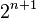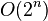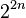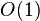# Space-time tradeoff facts for kids

Kids Encyclopedia Facts

In computer science, a space-time or time-memory tradeoff is a way of solving a problem or calculation in less time by using more storage space (or memory), or by solving a problem in very little space by spending a long time. Most computers have a large amount of space, but not infinite space. Also, most people are willing to wait a little while for a big calculation, but not forever. So if your problem is taking a long time but not much memory, a space-time tradeoff would let you use more memory and solve the problem more quickly. Or, if it could be solved very quickly but requires more memory than you have, you can try to spend more time solving the problem in the limited memory.

The most common condition is an algorithm using a lookup table. This means that the answers for some question for every possible value can be written down. One way of solving this problem is to write down the entire lookup table, which will let you find answers very quickly, but will use a lot of space. Another way is to calculate the answers without writing down anything, which uses very little space, but might take a long time.

A space-time tradeoff can be used with the problem of data storage. If data is stored uncompressed, it takes more space but less time than if the data were stored compressed (since compressing the data decreases the amount of space it takes, but it takes time to run the compression algorithm).

Larger code size can be used to increase program speed when using loop unwinding. This technique makes the program code longer for each iteration of a loop, but saves the computation time needed for jumping back to the beginning of the loop at the end of each iteration.

In the field of cryptography, using space-time tradeoff, the attacker is decreasing the exponential time required for a brute force attack. Rainbow tables use partially precomputed values in the hash space of a cryptographic hash function to crack passwords in minutes instead of weeks. Decreasing the size of the rainbow table increases the time required to iterate over the hash space. The meet-in-the-middle attack attack uses a space-time tradeoff to find the cryptographic key in only$2^{n+1}$ encryptions (and$O(2^n)$ space) compared to the expected$2^{2n}$ encryptions (but only$O(1)$ space) of the normal attack.

Dynamic programming is another example where the time of solving problems can be decreased by using more memory.In Spanish: Situación de compromiso espacio-tiempo para niños